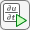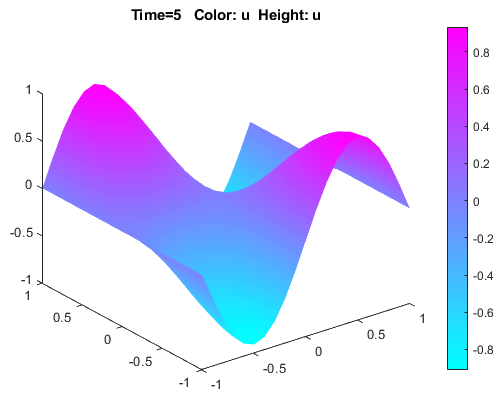## Wave Equation on Square Domain: PDE Modeler App

This example shows how to solve a wave equation for transverse vibrations of a membrane on a square. The membrane is fixed at the left and right sides, and is free at the upper and lower sides. This example uses the PDE Modeler app. For a programmatic workflow, see Wave Equation on Square Domain.

A wave equation is a hyperbolic PDE:

`$\frac{{\partial }^{2}u}{\partial {t}^{2}}-\Delta u=0$`

To solve this problem in the PDE Modeler app, follow these steps:

1. Open the PDE Modeler app by using the `pdeModeler` command.

2. Display grid lines by selecting Options > Grid.

3. Align new shapes to the grid lines by selecting Options > Snap.

4. Draw a square with the corners at (-1,-1), (-1,1), (1,1), and (1,-1). To do this, first click thebutton. Then click one of the corners using the right mouse button and drag to draw a square. The right mouse button constrains the shape you draw to be a square rather than a rectangle.

You also can use the `pderect` function:

`pderect([-1 1 -1 1])`
5. Check that the application mode is set to Generic Scalar.

6. Specify the boundary conditions. To do this, switch to boundary mode by clicking thebutton or selecting Boundary > Boundary Mode. Select the left and right boundaries. Then select Boundary > Specify Boundary Conditions and specify the Dirichlet boundary condition u = 0. This boundary condition is the default one (```h = 1```, `r = 0`), so you do not need to change it.

For the bottom and top boundaries, set the Neumann boundary condition u/∂n = 0. To do this, set `g = 0`, ```q = 0```.

7. Specify the coefficients by selecting PDE > PDE Specification or clicking thebutton on the toolbar. Select the Hyperbolic type of PDE, and specify ```c = 1```, `a = 0`, `f = 0`, and ```d = 1```.

8. Initialize the mesh by selecting Mesh > Initialize Mesh. Refine the mesh by selecting Mesh > Refine Mesh.

9. Set the solution times. To do this, select Solve > Parameters. Create linearly spaced time vector from 0 to 5 seconds by setting the solution time to `linspace(0,5,31)`.

10. In the same dialog box, specify initial conditions for the wave equation. For a well-behaved solution, the initial values must match the boundary conditions. If the initial time is t = 0, then the following initial values that satisfy the boundary conditions: `atan(cos(pi/2*x))` for `u(0)` and `3*sin(pi*x).*exp(sin(pi/2*y))` for u/∂t,

The inverse tangent function and exponential function introduce more modes into the solution.11. Solve the PDE by selecting Solve > Solve PDE or clicking thebutton on the toolbar. The app solves the heat equation at times from 0 to 5 seconds and displays the result at the end of the time span.

12. Visualize the solution as a 3-D static and animated plots. To do this:

1. Select Plot > Parameters.

2. In the resulting dialog box, select the Color and Height (3-D plot) options.

3. To visualize the dynamic behavior of the wave, select Animation in the same dialog box. If the animation progress is too slow, select the Plot in x-y grid option. An x-y grid can speed up the animation process significantly.